# Resources tagged with: Working systematically

Filter by: Content type:
Age range:
Challenge level:

### There are 132 results

Broad Topics > Thinking Mathematically > Working systematically### Games Related to Nim

##### Age 5 to 16

This article for teachers describes several games, found on the site, all of which have a related structure that can be used to develop the skills of strategic planning.### Window Frames

##### Age 5 to 14Challenge Level

This task encourages you to investigate the number of edging pieces and panes in different sized windows.### Maths Trails

##### Age 7 to 14

The NRICH team are always looking for new ways to engage teachers and pupils in problem solving. Here we explain the thinking behind maths trails.### LOGO Challenge - Triangles-squares-stars

##### Age 11 to 16Challenge Level

Can you recreate these designs? What are the basic units? What movement is required between each unit? Some elegant use of procedures will help - variables not essential.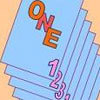### One Out One Under

##### Age 14 to 16Challenge Level

Imagine a stack of numbered cards with one on top. Discard the top, put the next card to the bottom and repeat continuously. Can you predict the last card?### Magic W

##### Age 14 to 16Challenge Level

Find all the ways of placing the numbers 1 to 9 on a W shape, with 3 numbers on each leg, so that each set of 3 numbers has the same total.### More Magic Potting Sheds

##### Age 11 to 14Challenge Level

The number of plants in Mr McGregor's magic potting shed increases overnight. He'd like to put the same number of plants in each of his gardens, planting one garden each day. How can he do it?### Olympic Magic

##### Age 14 to 16Challenge Level

in how many ways can you place the numbers 1, 2, 3 … 9 in the nine regions of the Olympic Emblem (5 overlapping circles) so that the amount in each ring is the same?### Triangles to Tetrahedra

##### Age 11 to 14Challenge Level

Imagine you have an unlimited number of four types of triangle. How many different tetrahedra can you make?### Cuboids

##### Age 11 to 14Challenge Level

Can you find a cuboid that has a surface area of exactly 100 square units. Is there more than one? Can you find them all?### Colour in the Square

##### Age 7 to 16Challenge Level

Can you put the 25 coloured tiles into the 5 x 5 square so that no column, no row and no diagonal line have tiles of the same colour in them?### Isosceles Triangles

##### Age 11 to 14Challenge Level

Draw some isosceles triangles with an area of $9$cm$^2$ and a vertex at (20,20). If all the vertices must have whole number coordinates, how many is it possible to draw?### Squares in Rectangles

##### Age 11 to 14Challenge Level

A 2 by 3 rectangle contains 8 squares and a 3 by 4 rectangle contains 20 squares. What size rectangle(s) contain(s) exactly 100 squares? Can you find them all?### Tetrahedra Tester

##### Age 11 to 14Challenge Level

An irregular tetrahedron is composed of four different triangles. Can such a tetrahedron be constructed where the side lengths are 4, 5, 6, 7, 8 and 9 units of length?### Shady Symmetry

##### Age 11 to 14Challenge Level

How many different symmetrical shapes can you make by shading triangles or squares?### Where Can We Visit?

##### Age 11 to 14Challenge Level

Charlie and Abi put a counter on 42. They wondered if they could visit all the other numbers on their 1-100 board, moving the counter using just these two operations: x2 and -5. What do you think?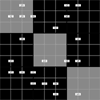### Ratio Sudoku 1

##### Age 11 to 16Challenge Level

A Sudoku with clues as ratios.### Neighbourly Addition

##### Age 7 to 14Challenge Level

I added together some of my neighbours' house numbers. Can you explain the patterns I noticed?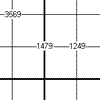### Another Quadruple Clue Sudoku

##### Age 11 to 16Challenge Level

This is a variation of sudoku which contains a set of special clue-numbers. Each set of 4 small digits stands for the numbers in the four cells of the grid adjacent to this set.### Problem Solving, Using and Applying and Functional Mathematics

##### Age 5 to 18Challenge Level

Problem solving is at the heart of the NRICH site. All the problems give learners opportunities to learn, develop or use mathematical concepts and skills. Read here for more information.### Charitable Pennies

##### Age 7 to 14Challenge Level

Investigate the different ways that fifteen schools could have given money in a charity fundraiser.### Gabriel's Problem

##### Age 11 to 14Challenge Level

Gabriel multiplied together some numbers and then erased them. Can you figure out where each number was?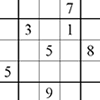### Corresponding Sudokus

##### Age 11 to 18

This second Sudoku article discusses "Corresponding Sudokus" which are pairs of Sudokus with terms that can be matched using a substitution rule.### Advent Calendar 2011 - Secondary

##### Age 11 to 18Challenge Level

Advent Calendar 2011 - a mathematical activity for each day during the run-up to Christmas.### A Long Time at the Till

##### Age 14 to 18Challenge Level

Try to solve this very difficult problem and then study our two suggested solutions. How would you use your knowledge to try to solve variants on the original problem?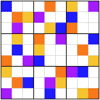### Ratio Sudoku 3

##### Age 11 to 16Challenge Level

A Sudoku with clues as ratios or fractions.### Diagonal Product Sudoku

##### Age 11 to 16Challenge Level

Given the products of diagonally opposite cells - can you complete this Sudoku?### Special Numbers

##### Age 11 to 14Challenge Level

My two digit number is special because adding the sum of its digits to the product of its digits gives me my original number. What could my number be?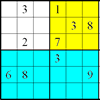### Colour Islands Sudoku

##### Age 11 to 14Challenge Level

An extra constraint means this Sudoku requires you to think in diagonals as well as horizontal and vertical lines and boxes of nine.### Multiply the Addition Square

##### Age 11 to 14Challenge Level

If you take a three by three square on a 1-10 addition square and multiply the diagonally opposite numbers together, what is the difference between these products. Why?### Summing Consecutive Numbers

##### Age 11 to 14Challenge Level

15 = 7 + 8 and 10 = 1 + 2 + 3 + 4. Can you say which numbers can be expressed as the sum of two or more consecutive integers?### Teddy Town

##### Age 5 to 14Challenge Level

There are nine teddies in Teddy Town - three red, three blue and three yellow. There are also nine houses, three of each colour. Can you put them on the map of Teddy Town according to the rules?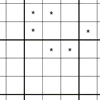### Addition Equation Sudoku

##### Age 11 to 14Challenge Level

You need to find the values of the stars before you can apply normal Sudoku rules.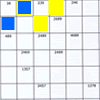### Intersection Sudoku 1

##### Age 11 to 16Challenge Level

A Sudoku with a twist.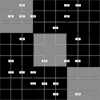### Ratio Sudoku 2

##### Age 11 to 16Challenge Level

A Sudoku with clues as ratios.### Sticky Numbers

##### Age 11 to 14Challenge Level

Can you arrange the numbers 1 to 17 in a row so that each adjacent pair adds up to a square number?### Spot the Card

##### Age 14 to 16Challenge Level

It is possible to identify a particular card out of a pack of 15 with the use of some mathematical reasoning. What is this reasoning and can it be applied to other numbers of cards?### Consecutive Negative Numbers

##### Age 11 to 14Challenge Level

Do you notice anything about the solutions when you add and/or subtract consecutive negative numbers?### Quadruple Clue Sudoku

##### Age 11 to 16Challenge Level

Four numbers on an intersection that need to be placed in the surrounding cells. That is all you need to know to solve this sudoku.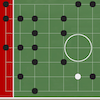### Phiddlywinks - a Tribute to John Conway

##### Age 7 to 18

Read this article to find out more about the inspiration for NRICH's game, Phiddlywinks.### Factor Lines

##### Age 7 to 14Challenge Level

Arrange the four number cards on the grid, according to the rules, to make a diagonal, vertical or horizontal line.### Instant Insanity

##### Age 11 to 18Challenge Level

Given the nets of 4 cubes with the faces coloured in 4 colours, build a tower so that on each vertical wall no colour is repeated, that is all 4 colours appear.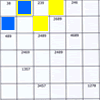### Intersection Sudoku 2

##### Age 11 to 16Challenge Level

A Sudoku with a twist.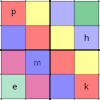### Simultaneous Equations Sudoku

##### Age 11 to 16Challenge Level

Solve the equations to identify the clue numbers in this Sudoku problem.### Two and Two

##### Age 11 to 16Challenge Level

How many solutions can you find to this sum? Each of the different letters stands for a different number.### Number Sandwiches

##### Age 7 to 14Challenge Level

Can you arrange the digits 1, 1, 2, 2, 3 and 3 to make a Number Sandwich?### 9 Weights

##### Age 11 to 14Challenge Level

You have been given nine weights, one of which is slightly heavier than the rest. Can you work out which weight is heavier in just two weighings of the balance?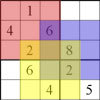### Twin Corresponding Sudoku

##### Age 11 to 18Challenge Level

This sudoku requires you to have "double vision" - two Sudoku's for the price of one### The Great Weights Puzzle

##### Age 14 to 16Challenge Level

You have twelve weights, one of which is different from the rest. Using just 3 weighings, can you identify which weight is the odd one out, and whether it is heavier or lighter than the rest?### Read This Page

##### Age 11 to 14Challenge Level

Replace the letters with numbers to make the addition work out correctly. R E A D + T H I S = P A G E